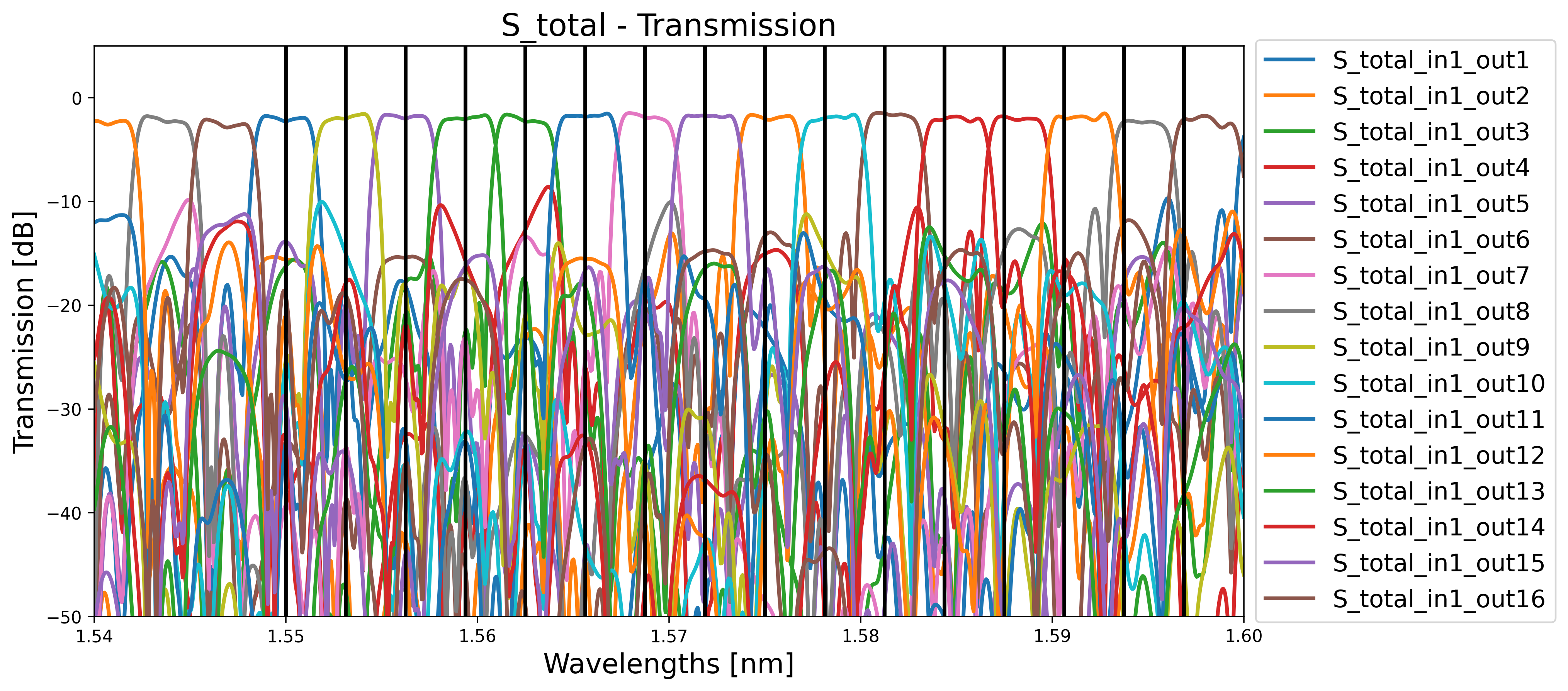# 1.3. Four-way WDM¶

As a next step, we increase the complexity of the demultiplexer. We create a four-way wavelength demultiplexer with a passband of 25% of the FSR at the provided center wavelength. It is implemented by staging a two-way demultiplexer on half the FSR, followed by two two-way demultiplexers tuned to the frequencies coming out of the first stage. The operating principle is shown in the figure below. The first stage (S1) splits the odd- and even-numbered frequency channels. In the second stage, the MUX2 at the bottom (S2 down) splits the two incoming odd-numbered channels, while the MUX2 at the top (S2 up) splits the even ones.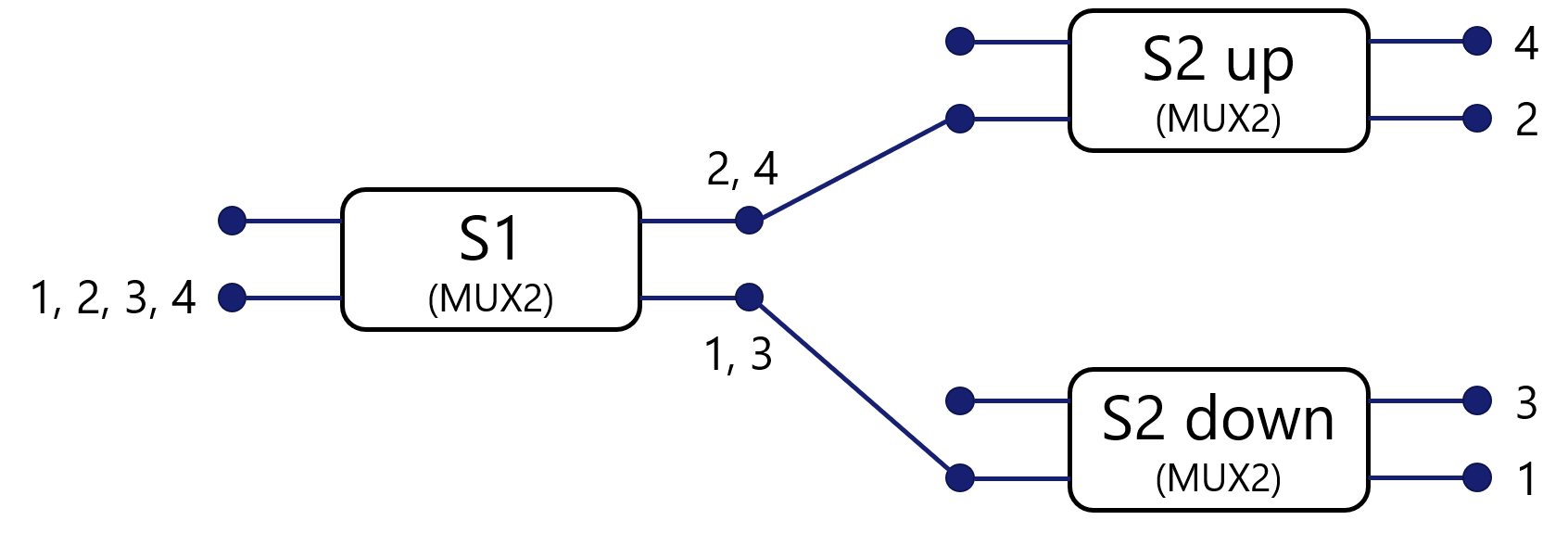We define a class Mux4 that inherits from CircuitCell and uses Mux2 as default PCell for the first and the second stage.

class Mux4(CircuitCell):
"""Four-way wavelength demultiplexer with a passband of 25% of the FSR at the center_wavelength.
It is implemented by staging a two-way demultiplexer on half the FSR, followed by two two-way
de-multiplexers tuned to the frequencies coming out of the first stage.
"""
spacing_x = i3.PositiveNumberProperty(default=100.0, doc="Port-to-port spacing between the MUXs in the x-direction")
spacing_y = i3.PositiveNumberProperty(default=150.0, doc="Port-to-port spacing between the MUXs in the y-direction")
fsr = i3.PositiveNumberProperty(default=0.02, doc="Free spectral range of the MUX4")
center_wavelength = i3.PositiveNumberProperty(default=1.55, doc="Center wavelength")
stage_1 = i3.ChildCellProperty(doc="MUX2 for the first stage")
stage_2_up = i3.ChildCellProperty(doc="MUX2 for the second stage (up)")
stage_2_down = i3.ChildCellProperty(doc="MUX2 for the second stage (down)")

def _default_stage_1(self):
return Mux2(center_wavelength=self.center_wavelength,
fsr=self.fsr / 2.0,
name=self.name + "_stage1")

def _default_stage_2_up(self):
return Mux2(center_wavelength=self.center_wavelength + self.fsr / 4,
fsr=self.fsr,
name=self.name + "_stage2_up")

def _default_stage_2_down(self):
return Mux2(center_wavelength=self.center_wavelength,
fsr=self.fsr,
name=self.name + "_stage2_down")

def _default_child_cells(self):
child_cells = dict()
child_cells["mux_0_0"] = self.stage_1
child_cells["mux_1_0"] = self.stage_2_down
child_cells["mux_1_1"] = self.stage_2_up
return child_cells

def _default_place_specs(self):
x = self.spacing_x
y = self.spacing_y
place_specs = [i3.Place("mux_0_0", (0, 0)),
i3.PlaceRelative("mux_1_0:in1", "mux_0_0:out1", (x, -y / 2)),
i3.PlaceRelative("mux_1_1:in1", "mux_0_0:out2", (x, y / 2))]
return place_specs

def _default_connectors(self):
conn = []
conn.append(("mux_0_0:out1", "mux_1_0:in1", bezier_sbend))
conn.append(("mux_0_0:out2", "mux_1_1:in1", bezier_sbend))
return conn

def _default_external_port_names(self):
epn = dict()
epn["mux_0_0:in1"] = "in1"
epn["mux_0_0:in2"] = "in2"
epn["mux_1_0:out1"] = "out1"
epn["mux_1_0:out2"] = "out2"
epn["mux_1_1:out1"] = "out3"
epn["mux_1_1:out2"] = "out4"
return epn


Two more properties are added to Mux4 compared to Mux2 and can be adapted by the user:

• spacing_x: the port-to-port spacing between the demultiplexers in the x-direction.
• spacing_y: the port-to-port spacing between the demultiplexers in the y-direction.

As for the two-way demultiplexer, we can visualize the layout and the circuit simulation:

1. Layout:

    # Writing the layout
cell = Mux4(name="MUX4",
fsr=0.05,
center_wavelength=1.55,
spacing_x=50,
spacing_y=80)
cell_lv = cell.Layout()
cell_lv.visualize(annotate=True)
cell_lv.write_gdsii("mux4.gds")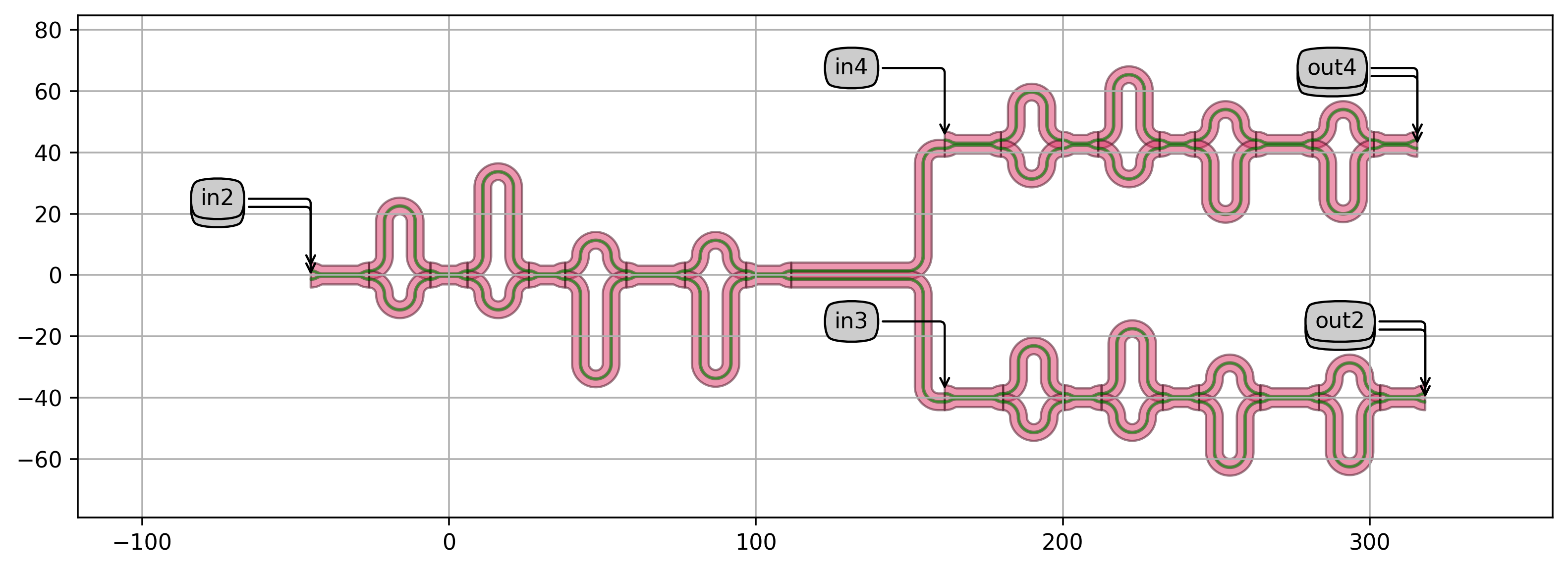2. Transmission spectrum of the first stage: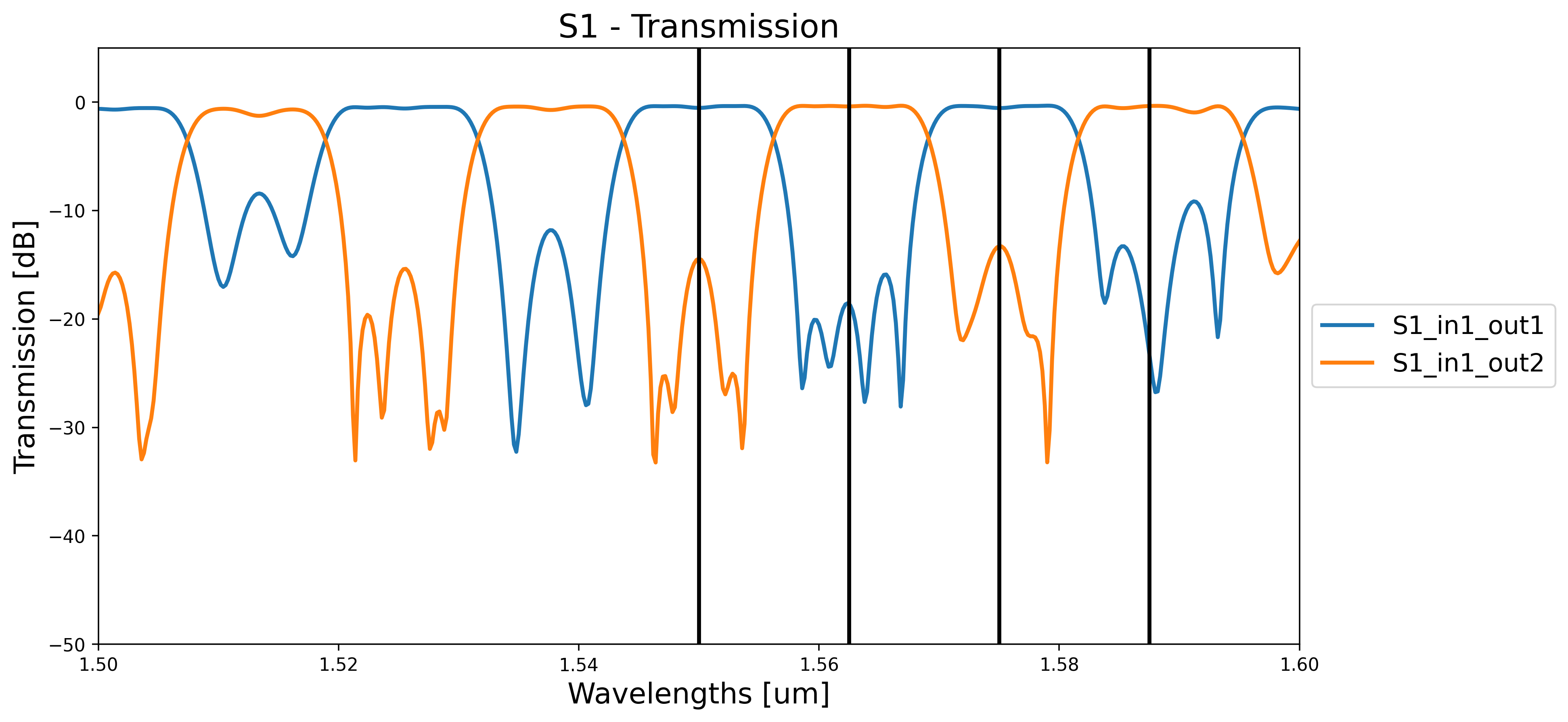3. Transmission spectrum of the second stage, bottom branch: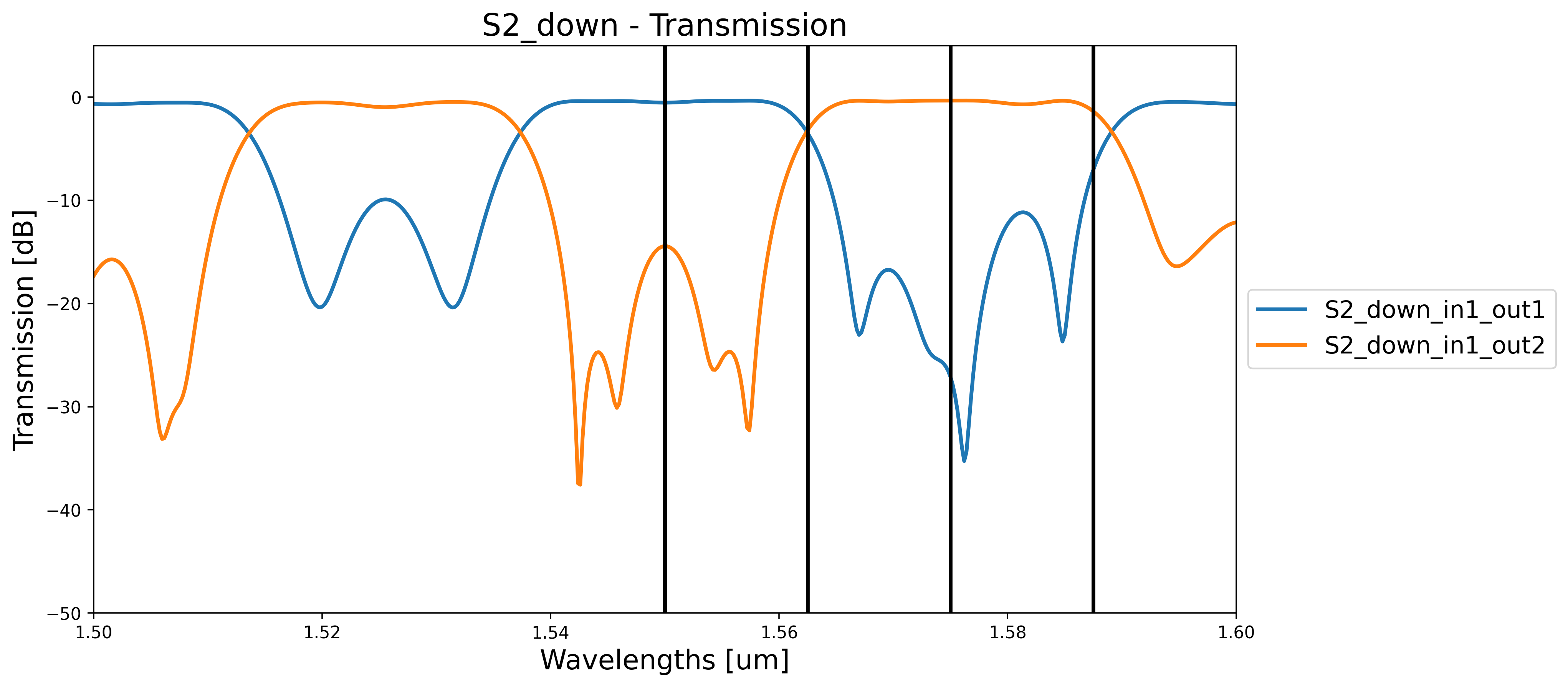4. Transmission spectrum of the second stage, top branch: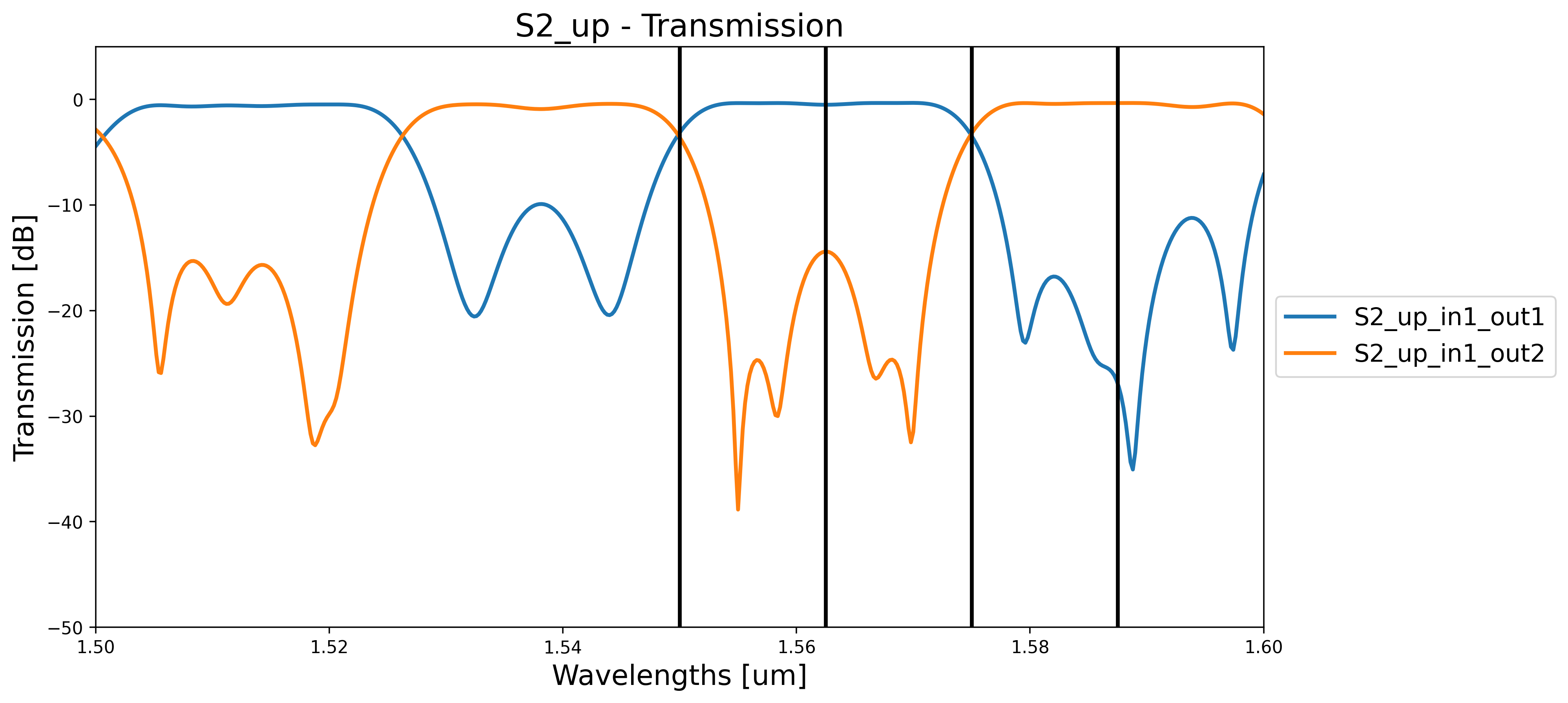5. Total transmission spectrum of the MUX4: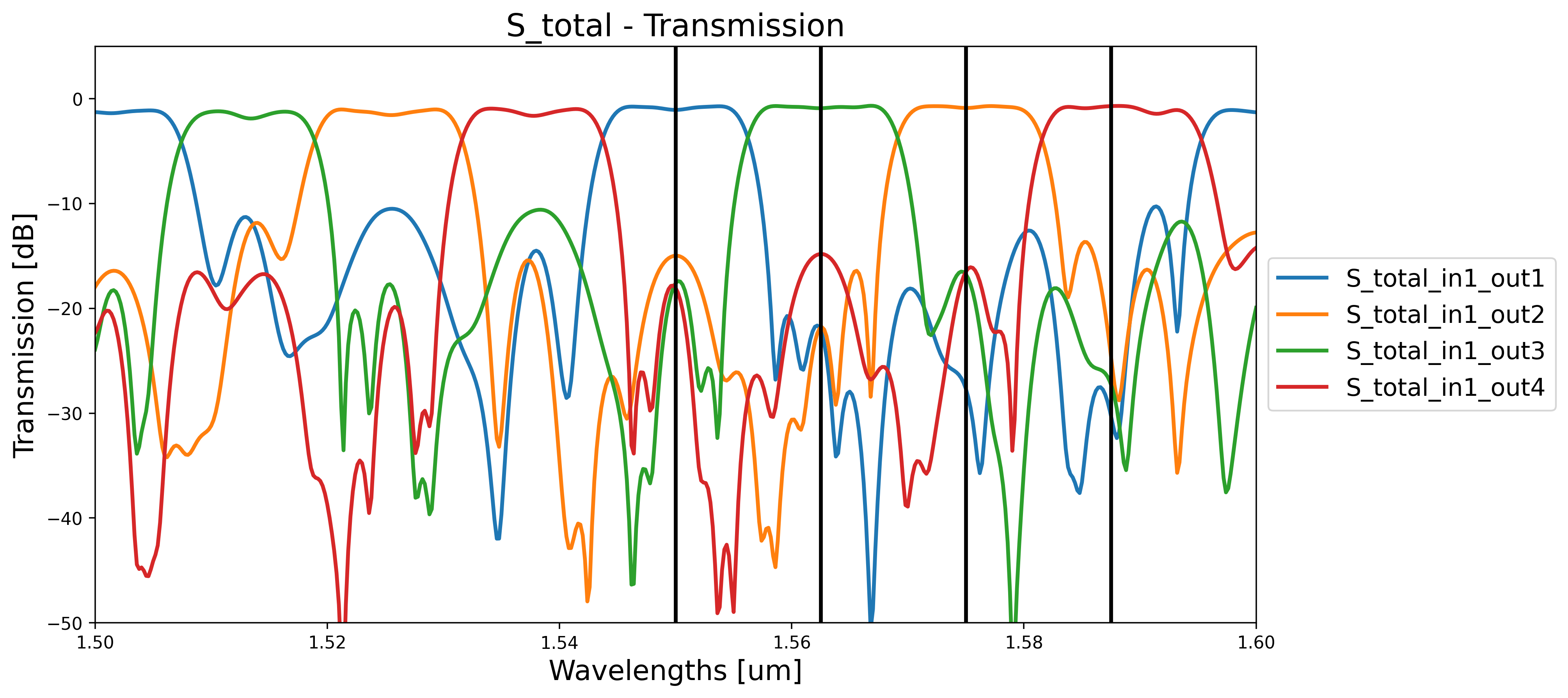# 1.4. Eight-way WDM¶

Similarly, we can now create an eight-way demultiplexer with a passband of 12.5% of the FSR at the provided center wavelength. It is implemented by staging a four-way demultiplexer on half the FSR, followed by four two-way demultiplexers tuned to the frequencies coming out of the first stage. The operating principle is shown in the figure below. In the first stage (S1), the MUX4 splits the incoming frequencies in four pairs (1-4, 3-7, 2-6, 4-8). In the second stage, we use four MUX2 (S2_0, S2_1, S2_2, S2_3) to split in two each pair of frequencies coming out of the first stage.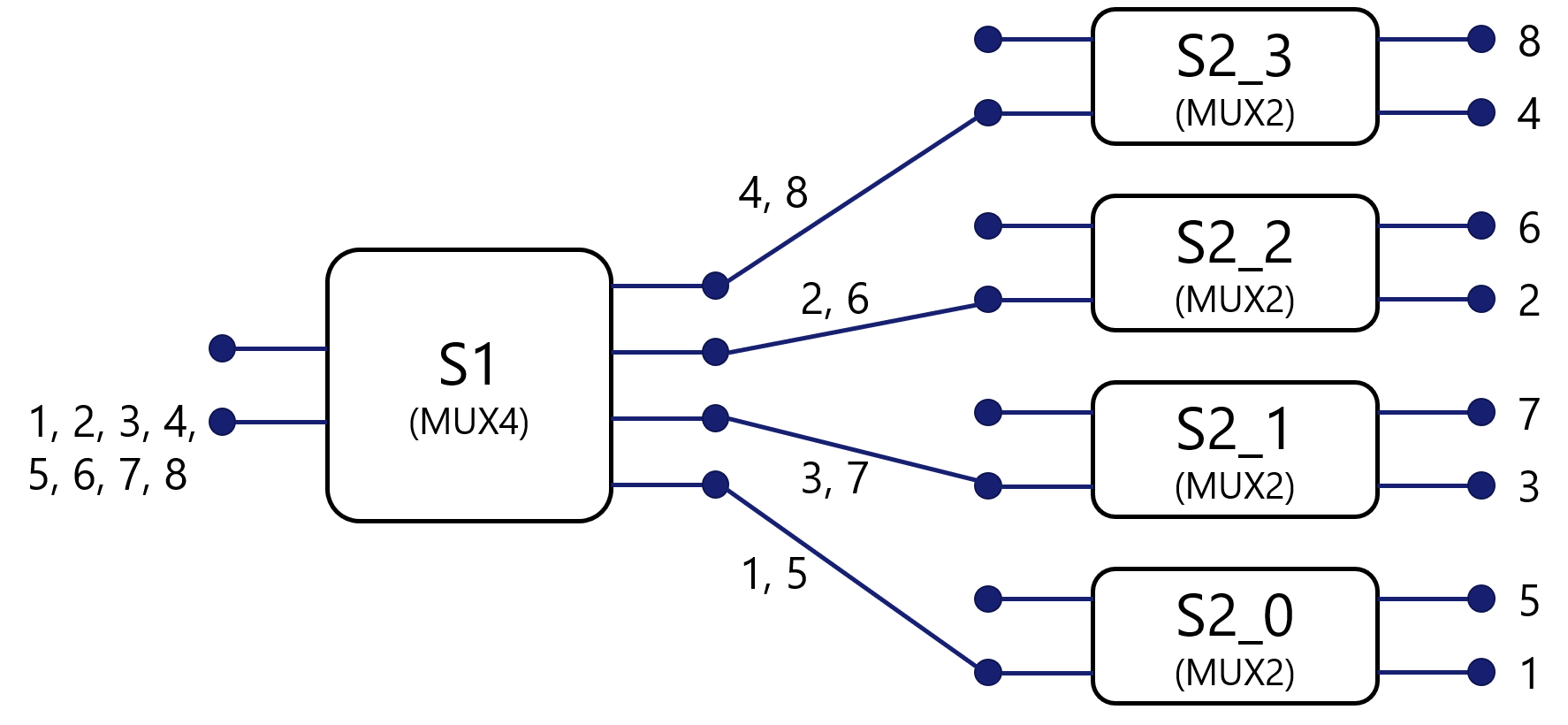We define a class Mux4 that inherits from CircuitCell. It uses Mux4 as default PCell for the first stage and Mux2 for the second stage.

class Mux8(CircuitCell):
"""Eight-way wavelength demultiplexer with a passband of 12.5% of the FSR at the center_wavelength.
It is implemented by staging a four-way multiplexer on half the FSR, followed by four two-way
demultiplexers tuned to the frequencies coming out of the first stage.
"""
spacing_x = i3.PositiveNumberProperty(default=150.0, doc="Port-to-port spacing between the MUXs in the x-direction")
spacing_y = i3.PositiveNumberProperty(default=200.0, doc="Port-to-port spacing between the MUXs in the y-direction")
fsr = i3.PositiveNumberProperty(default=0.01, doc="Free spectral range of the MUX8")
center_wavelength = i3.PositiveNumberProperty(default=1.55, doc="Center wavelength")
stage_1 = i3.ChildCellProperty(doc="MUX4 for the first stage")
stage_2 = i3.ChildCellListProperty(doc="MUX2 for the second stages")

def _default_stage_1(self):
return Mux4(center_wavelength=self.center_wavelength,
fsr=self.fsr / 2.0,
name=self.name + "_stage1",
spacing_x=self.spacing_x,
spacing_y=self.spacing_y)

def _default_stage_2(self):
stages = []
channels = [1, 3, 2, 4]
for cnt, channel in enumerate(channels):
cell = Mux2(center_wavelength=self.center_wavelength + (channel - 1) * self.fsr / 8.0,
fsr=self.fsr,
name=self.name + "_stage2_{}".format(cnt))
stages.append(cell)
return stages

def _default_child_cells(self):
child_cells = dict()
child_cells["mux_0_0"] = self.stage_1
for cnt, stage in enumerate(self.stage_2):
child_cells["mux_1_{}".format(cnt)] = stage
return child_cells

def _default_place_specs(self):
x = self.spacing_x
y = self.spacing_y
x0 = self.stage_1.get_default_view(i3.LayoutView).size_info().east

place_specs = [i3.Place("mux_0_0", (0, 0))]
for cnt in range(4):
place_specs.append(i3.Place("mux_1_{}:in1".format(cnt), (x0 + x, (-3 + 2 * cnt) * y / 4.0)))
return place_specs

def _default_connectors(self):
conn = []
for cnt in range(4):
conn.append(("mux_0_0:out{}".format(cnt + 1), "mux_1_{}:in1".format(cnt), bezier_sbend))
return conn

def _default_external_port_names(self):
epn = dict()
epn["mux_0_0:in1"] = "in1"
epn["mux_0_0:in2"] = "in2"
for cnt in range(4):
epn["mux_1_{}:out1".format(cnt)] = "out{}".format(2 * cnt + 1)
epn["mux_1_{}:out2".format(cnt)] = "out{}".format(2 * cnt + 2)
return epn


We can now visualize the layout and the circuit simulation:

1. Layout:

    # Writing the layout
cell = Mux8(name="MUX8",
fsr=0.02,
center_wavelength=1.55)
cell_lv = cell.Layout()
cell_lv.visualize(annotate=True)
cell_lv.write_gdsii("mux8.gds")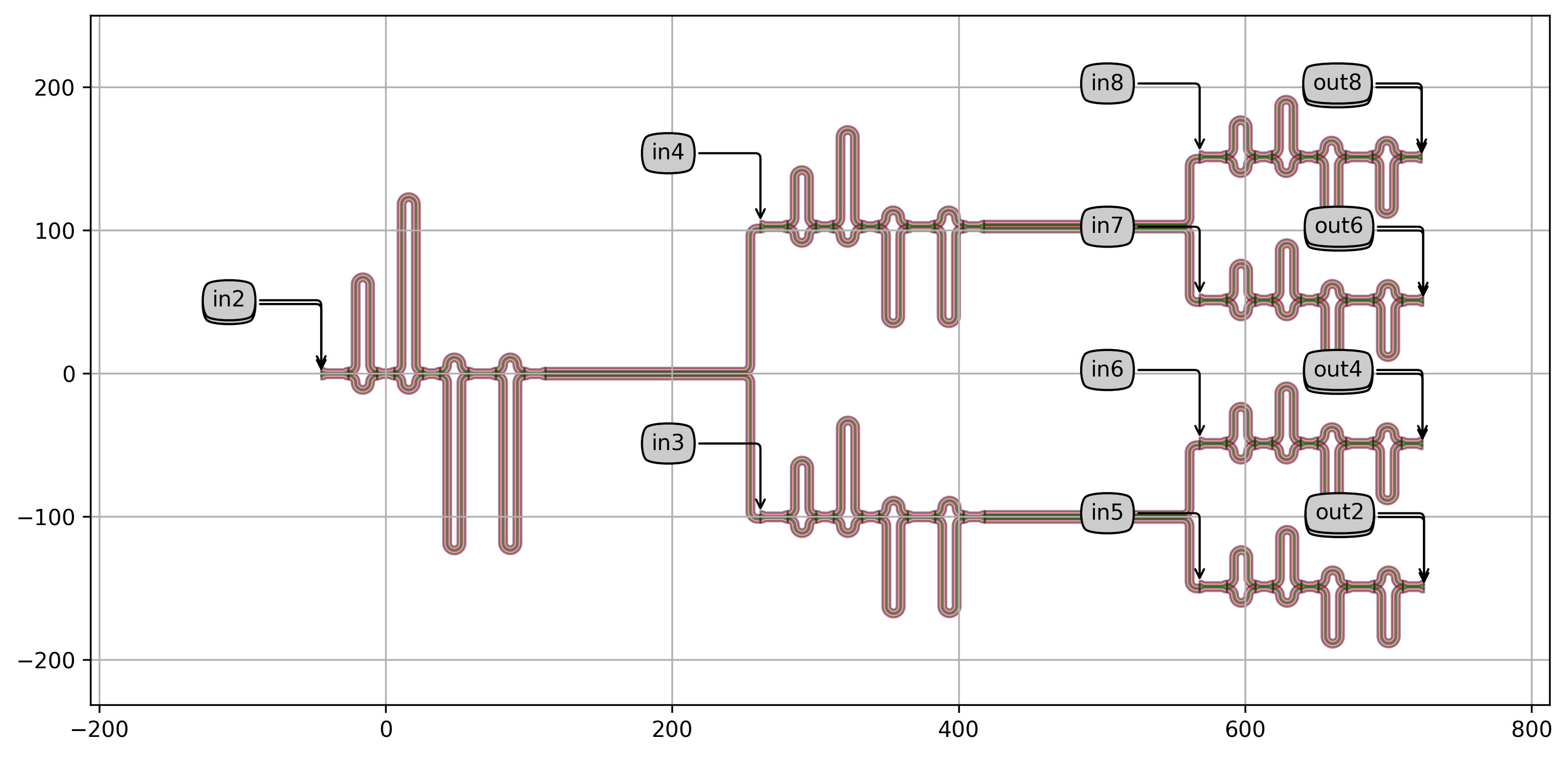2. Total transmission spectrum of the MUX8: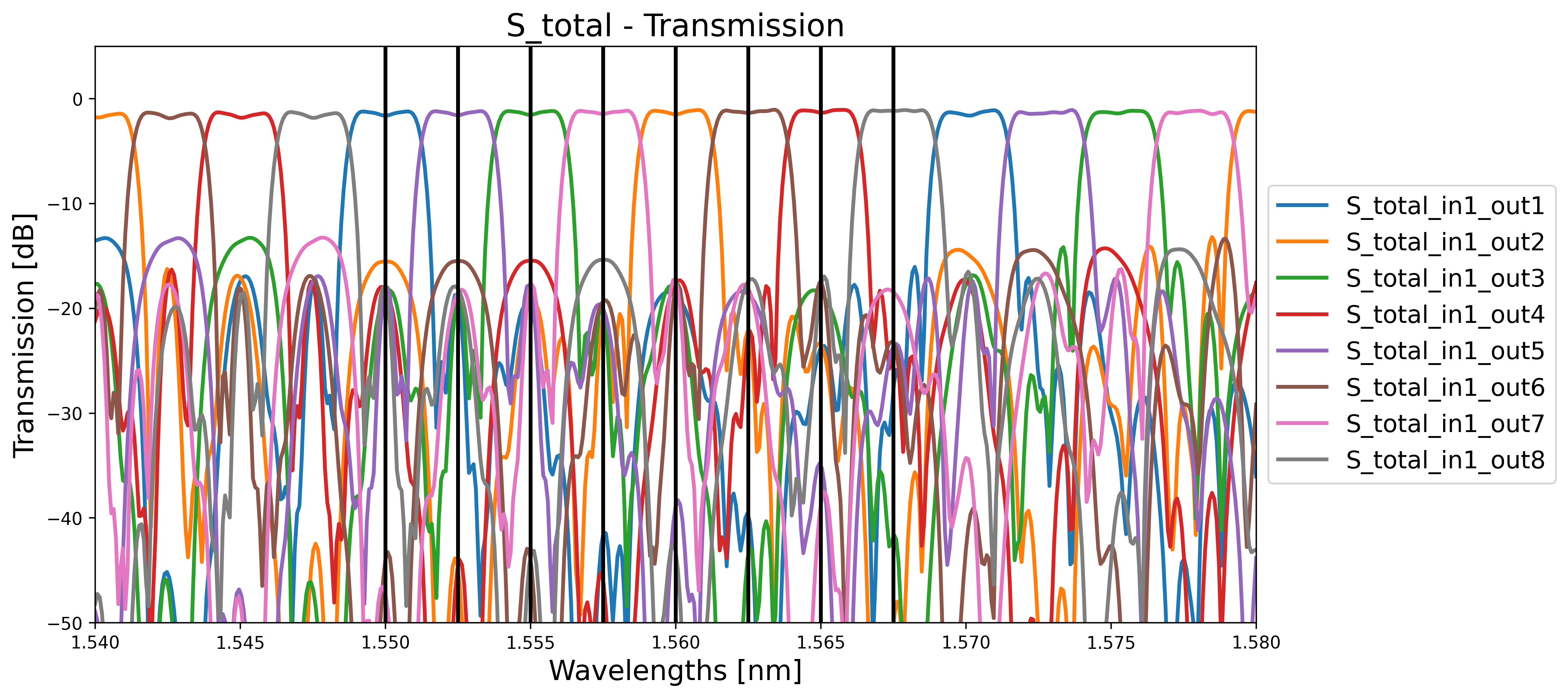# 1.5. Parametric WDM¶

Finally, following the same logic used to define Mux4 and Mux8, we create a parametric wavelength demultiplexer. If $$n$$ is the number of stages, then the demultiplexer will have $$2^{n+1}$$ channels and a passband of $$100/(2^{n+1})$$ that inherits from CircuitCell. It is implemented by staging a ($$n-1$$)-way demultiplexer on half the FSR, followed by $$n$$ two-way demultiplexers (Mux2) tuned to the frequencies coming out of the first stage.

class MuxParametric(CircuitCell):
"""2**(n+1)-way wavelength demultiplexer with a passband of 100/2**(n+1)% of the FSR at the center_wavelength.
It is implemented by staging a n-1-way demultiplexer on half the FSR, followed by n two-way
demultiplexers tuned to the frequencies coming out of the first stage.
"""
spacing_x = i3.PositiveNumberProperty(default=250.0, doc="Port-to-port spacing between the MUXs in the x-direction")
spacing_y = i3.PositiveNumberProperty(default=200.0, doc="Port-to-port spacing between the MUXs in the y-direction")
fsr = i3.PositiveNumberProperty(default=0.01, doc="Free spectral range of the MUX with N stages")
n_stages = i3.IntProperty(default=3, doc="Number of stages")
center_wavelength = i3.PositiveNumberProperty(default=1.55, doc="Center wavelength")
phase_error_width_deviation = i3.NonNegativeNumberProperty(default=0.0)
phase_error_correlation_length = i3.NonNegativeNumberProperty(default=0.0)
stage_1 = i3.ChildCellProperty(doc="MUX with N stages for the first stage")
stage_2 = i3.ChildCellListProperty(doc="MUX2 for the second stages")

def n_channels(self):
return int(2 ** (self.n_stages + 1))

def _default_stage_1(self):
if self.n_stages > 0:
cell = MuxParametric(name=self.name + "_stage1",
center_wavelength=self.center_wavelength,
fsr=self.fsr / 2.0,
n_stages=self.n_stages - 1,
spacing_x=self.spacing_x,
spacing_y=self.spacing_y * 2,
phase_error_width_deviation=self.phase_error_width_deviation,
phase_error_correlation_length=self.phase_error_correlation_length)
else:
cell = Mux2(name=self.name + "_stage1",
center_wavelength=self.center_wavelength,
fsr=self.fsr,
phase_error_width_deviation=self.phase_error_width_deviation,
phase_error_correlation_length=self.phase_error_correlation_length)
return cell

def _default_stage_2(self):
stages = []

for cnt in range(self.n_channels() / 2):
cell = Mux2(name=self.name + "_stage2_{}".format(cnt),
center_wavelength=self.center_wavelength + cnt * self.fsr / self.n_channels(),
fsr=self.fsr,
phase_error_width_deviation=self.phase_error_width_deviation,
phase_error_correlation_length=self.phase_error_correlation_length)
stages.append(cell)
return stages

def _default_child_cells(self):
children = dict()
children["mux_0_0"] = self.stage_1
if self.n_stages > 0:
for cnt, stage in enumerate(self.stage_2):
children["mux_1_{}".format(cnt)] = stage
return children

def _default_place_specs(self):
place_specs = []
place_specs.append(i3.Place("mux_0_0",  (0, 0)))

if self.n_stages > 0:
x = self.spacing_x
y = self.spacing_y
x0 = self.stage_1.get_default_view(i3.LayoutView).size_info().east
tot_y = (self.n_channels() / 2 - 1) * y
for cnt in range(self.n_channels() / 2):
place_specs.append(i3.Place("mux_1_{}:in1".format(cnt), (x0 + x, -tot_y / 2.0 + cnt * y)))
return place_specs

def _default_connectors(self):
conn = []
if self.n_stages > 0:
for cnt in range(self.n_channels() / 2):
conn.append(("mux_0_0:out{}".format(cnt + 1), "mux_1_{}:in1".format(cnt), manhattan,
return conn

def _default_external_port_names(self):
epn = dict()
epn["mux_0_0:in1"] = "in1"
epn["mux_0_0:in2"] = "in2"
if self.n_stages > 0:
for cnt in range(self.n_channels() / 2):
epn["mux_1_{}:out1".format(cnt)] = "out{}".format(2 * cnt + 1)
epn["mux_1_{}:out2".format(cnt)] = "out{}".format(2 * cnt + 2)
else:
epn["mux_0_0:out1"] = "out1"
epn["mux_0_0:out2"] = "out2"
return epn


We can now visualize the layout and the circuit simulation:

1. Layout:

    # Writing the layout
cell = MuxParametric(name="MUX",
n_stages=3,
fsr=0.05,
center_wavelength=1.55,
spacing_x=40,
spacing_y=60,
phase_error_width_deviation=0.001,
phase_error_correlation_length=1.0)
cell_lv = cell.Layout()
cell_lv.visualize(annotate=True)
cell_lv.write_gdsii("mux{}.gds".format(cell.n_channels()))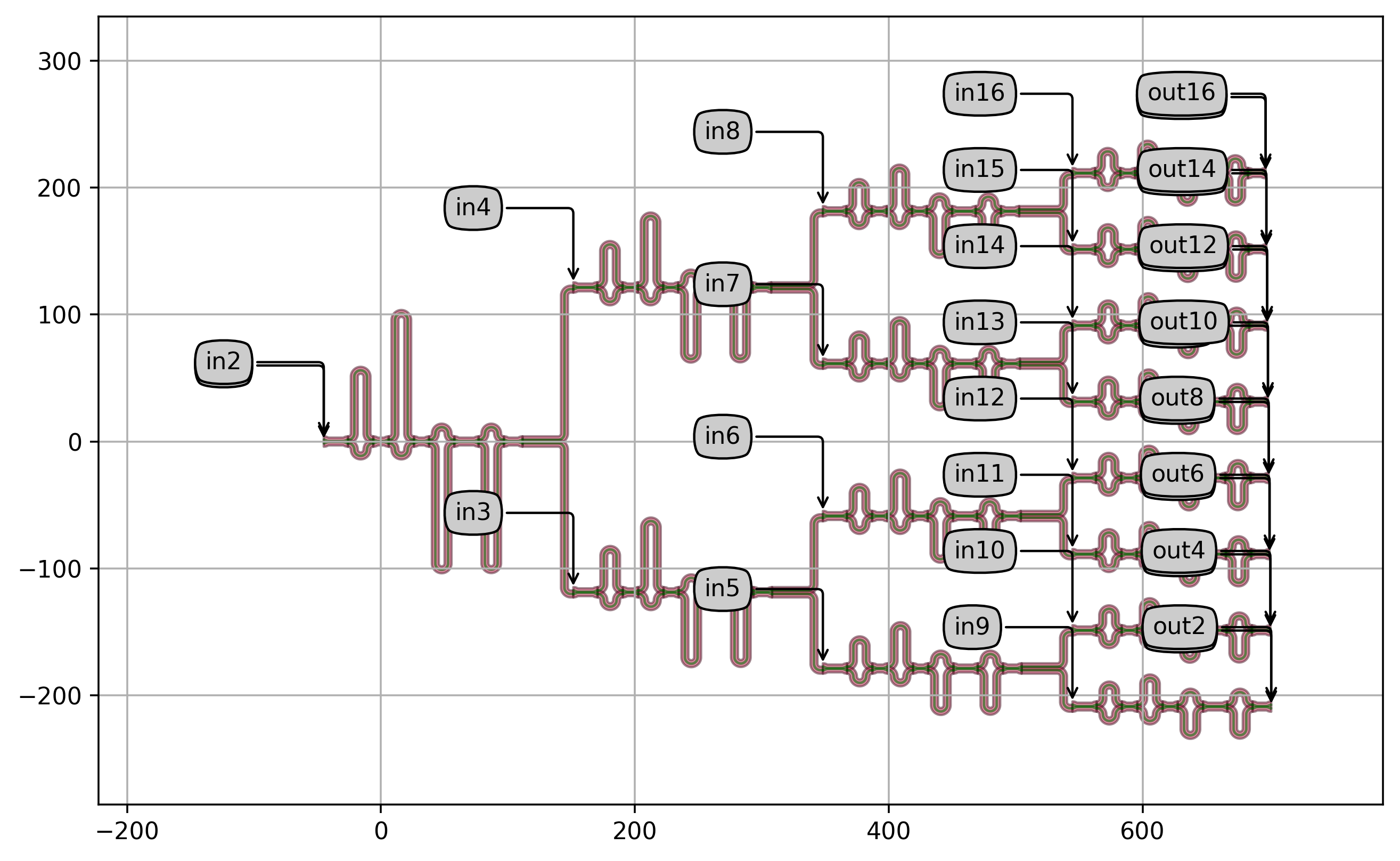2. Total transmission of the CWDM: